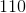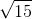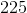# PSAT Math : How to multiply exponents

## Example Questions

### Example Question #11 : Exponents

(x3)2 * x2

x2

x4

x6

x

x4

x4

Explanation:

When an exponent is raised to a power, we multiply. But when two exponents with the same base are multiplied, we add them. So (x3)2 = x3*2 = x6. Then (x3)2 * x2 = x6 * x2 = x6 – 2 = x4.

### Example Question #11 : Exponents

If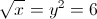, then which of the following is equivalent to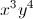?

6^7

6^4

6^8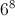6^6Explanation:

We can break up the equation into two smaller equations involving only x and y. Then, once we solve for x and y, we can find the value of.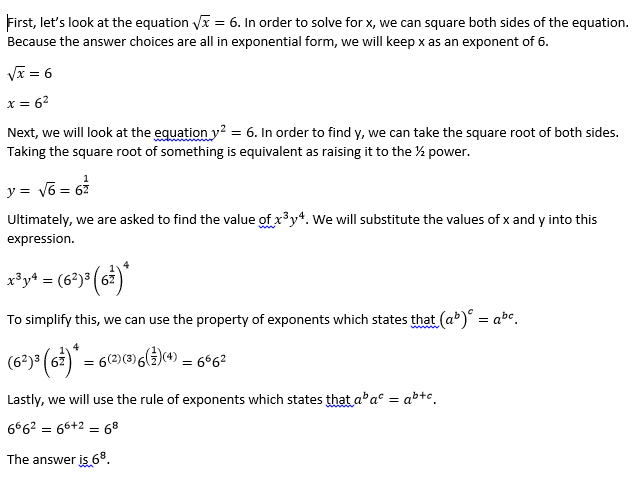### Example Question #41 : Exponents

If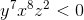which of the following must be true?

I.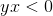II.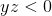III.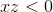I

III

None

All

II

None

Explanation:must be negative because it has an odd power andandhave even powers above. Butandcould be positive or negative, so none of the scenarios has to be true.

### Example Question #11 : How To Multiply Exponents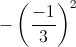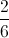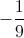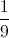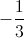Explanation: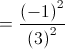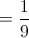Hence the correct answer will be### Example Question #11 : Exponents

Solve for: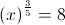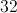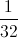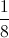Explanation:

IfThen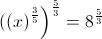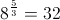and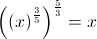Hence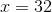### Example Question #51 : Exponents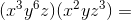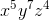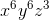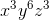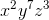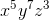Explanation:

(x3y6z)(x2yz3)

The paraentheses are irrelevant. Rearrange to combine like terms.

x3x2y6y1z1z3

When you multiply variables with exponents, simply add the exponents together.

x3+2 y6+1 z1+3

x5y7z4

### Example Question #51 : Exponents

If an original bacteria colony contains six organisms, and triples every hour, how many organisms are there after 7 hours?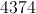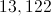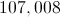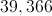Explanation:

To find the answer we can apply the equation of population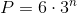whereis the number of hours.

### Example Question #491 : Algebra

Simplify: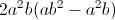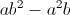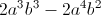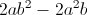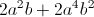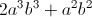Explanation:

Use the distributive property:.  When we multiply variables with exponents, we keep the same base and add the exponents: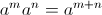### Example Question #12 : Exponents

Simplify: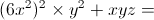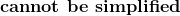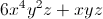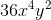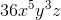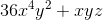Explanation: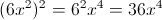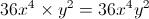We cannot combine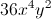with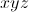, so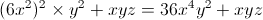.

### Example Question #492 : Algebra

If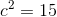, then what is the value of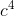?